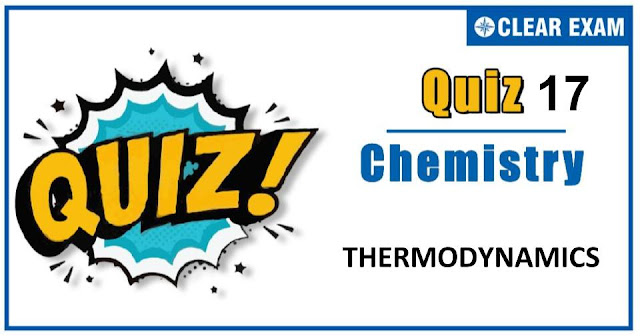## Thermodynamics Quiz-17

Thermodynamics is a very important branch of both chemistry and physics. It deals with the study of energy, the conversion of energy between different forms and the ability of energy to do work. Thermodynamics is an important chapter for JEE Mains, JEE Advance NEET and many others exams. As this chapter is present in both chemistry and physics and there is only a minor difference between them hence it becomes more important topic. It is not a tough topic, it only requires practice. This topic has been given good weightage in all engineering exams. So don't skip this and prepare well. All the best !.

Q1. Thermochemistry is the study of relationship between heat energy and :
•  Chemical energy
•  Activation energy
•  Frictional energy
•  None of these
Solution:-
The branch deals with interconversion of heat and chemical energy.

Q2. If gas, at constant temperature and pressure expands then its
•  Entropy increases and then decreases
•  Internal energy increases
•  Internal energy remains the same
•  Internal energy decreases
Solution:-
Internal energy of a gas depends upon its pressure and temperature. Thus, if a gas expands at constant temperature and pressure, then its internal energy remains same.

Q3. The total amount of energy in the universe is fixed, but:
•  Matter is increasing
•  Gravitation is decreasing
•  Disorder is increasing
•  Lightening is increasing
Solution:-
Entropy (a measure of disorder) of universe is increasing towards maximum. This is second law of thermodynamics.

Q4. ΔE° of combustion of isobutylene is −x kJ/mol. The value of ΔH° is :
•  = ΔE°
•  > ΔE°
•  = 0
•  < ΔE°
Solution:-
(CH3)2C=CH2(g) + 6O2(g) → 4CO2(g) + 4H2O(l)
Δng = 4−7 = −3
We know that ΔH = ΔE + ΔngRT
= ΔE − (Δm)RT   (∵ Δng = −ve)
∴ ΔH < ΔE

Q5. The work done during the process when 1 mole of gas is allowed to expand freely into vacuum is:
•  Zero
•  +ve
•  −ve
•  Either of these
Solution:-
W = −Pext.(V2−V1)
∵   Pext. = 0
∴   W = 0

Q6. The heat of combustion of rhombic and monoclinic sulphur are 70.96 and 71.03 kcal. The heat of transition of SR→M is:
•  70.96 kcal
•  71.03 kcal
•  −70 cal
•  +70 cal
Solution:-
SR + O2 → SO2; ΔH = −70.96 kcal.....(i)
SM + O2 → SO2; ΔH = −71.03 kcal.....(ii)
By eq. (i) − (ii)
SR → SM; ΔH = 0.07 kcal or 70 cal

Q7. From the following bond energies :
H−H bond energy : 431.37 kJ/mol
C=C bond energy : 606.10 kJ/mol
C−C bond energy : 336.49 kJ/mol
C−H bond energy : 410.50 kJ/mol
Enthalpy for the reaction,
•  553.0 kJ/mol
•  1523.6 kJ/mol
•  −243.6 kJ/mol
•  −120.0 kJ/mol
Solution:-

Q8. 1 mole of gas occupying 3 L volume is expanded against a constant external pressure of 1 atm to a volume of 15 litre. The work done by the system is:
•  1.215 × 103 J
•  12.15 × 103 J
•  121.5 × 103 J
•  None of these
Solution:-
−Wirr. = Pext.(V2−V1)
= 1 × (15−3) = 12 litre atm
= (12 × 1.987 × 4.184) / 0.0821 = 1.215 × 103 J

Q9. Which one of the following is not a state function?
•  Enthalpy
•  Entropy
•  Work
•  Free energy
Solution:-
Macroscopic properties which determine the state of a system are referred as state functions. The change in the state properties depends only upon the initial and final state of the system. All thermodynamic functions are state functions except work and heat.

Q10. A closed flask contains water in all its three states, solids, liquid and vapour at 0°C. In this situation the average KE of the water molecule will be:
•  Maximum in vapour state
•  Maximum in solid state
•  Greater in the liquid than in vapour state
•  Same in all the three states
Solution:-
KE = (3/2)RT## Want to know more

Please fill in the details below:

## Latest NEET Articles\$type=three\$c=3\$author=hide\$comment=hide\$rm=hide\$date=hide\$snippet=hide

Name

ltr
item
BEST NEET COACHING CENTER | BEST IIT JEE COACHING INSTITUTE | BEST NEET & IIT JEE COACHING: Thermodynamics Quiz-17
Thermodynamics Quiz-17
https://1.bp.blogspot.com/-oGz8yvPuRrI/X5l0QQmC9qI/AAAAAAAAB4w/jveX_1fKgXIgu5p0R3AAe8s7-Tjv2wgNgCLcBGAsYHQ/w640-h336/Quiz%2BImage%2B20%2B%252813%2529.jpg
https://1.bp.blogspot.com/-oGz8yvPuRrI/X5l0QQmC9qI/AAAAAAAAB4w/jveX_1fKgXIgu5p0R3AAe8s7-Tjv2wgNgCLcBGAsYHQ/s72-w640-c-h336/Quiz%2BImage%2B20%2B%252813%2529.jpg
BEST NEET COACHING CENTER | BEST IIT JEE COACHING INSTITUTE | BEST NEET & IIT JEE COACHING
https://www.cleariitmedical.com/2020/10/thermodynamics-quiz-7.html
https://www.cleariitmedical.com/
https://www.cleariitmedical.com/
https://www.cleariitmedical.com/2020/10/thermodynamics-quiz-7.html
true
7783647550433378923
UTF-8

STAY CONNECTED